Algebra (Mathematics) Class 6 - NCERT Questions
Q 1.

Find the rule which gives the number of matchsticks required to make the following matchstick patterns. Use a variable to write the rule.
(A) A pattern of letter T as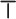(B) A pattern of letter Z as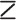(C) A pattern of letter U as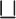(D) A pattern of letter V as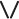(E) A pattern of letter E as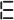(F) A pattern of letter S as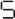(G) A pattern of letter A as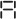SOLUTION:

(A) Number of matchsticks required to make one= 2
∴ Number of matchsticks required to make a pattern of letter T as= 2n
(B) Number of matchsticks required to make one= 3
∴ Number of matchsticks required to make a pattern of letter Z as= 3n
(C) Number of matchsticks required to make one= 3
∴ Number of matchsticks required to make a pattern of letter U as= 3n
(D) Number of matchsticks required to make one= 2
∴ Number of matchsticks required to make a pattern of letter V as= 2n
(E) Number of matchsticks required to make one= 5
∴ Number of matchsticks required to make a pattern of letter E as= 5n
(F) Number of matchsticks required to make one= 5
∴ Number of matchsticks required to make a pattern of letter S as= 5n
(G) Number of matchsticks required to make one= 6
∴ Number of matchsticks required to make a pattern of letter A as= 6n

Q 2.

We already know the rule for the pattern of letters L, C and F. Some of the letters from Q.1 (given above) gives us the same rule as that given by L. Which are these?
Why does this happen?

SOLUTION:

Part (A) & (D) i.e., letter T and V have same rule as that given by L because the number of matchsticks required in each of them is 2.

Q 3.

5 cadets in a row. What is the rule which gives the number of cadets, given the number of rows? (Use n for the number of rows.)

SOLUTION:

Let number of rows = n
Number of cadets in each row = 5
Therefore, total number of cadets = 5n

Q 4.

If there are 50 mangoes in a box, how will you write the total number of mangoes in terms of the number of boxes? (Use b for the number of boxes.)

SOLUTION:

Let number of boxes = b
Number of mangoes in each box = 50
Therefore, total number of mangoes = 50b

Q 5.

The teacher distributes 5 pencils per student. Can you tell how many pencils are needed, given the number of students? (Use s for the number of students.)

SOLUTION:

Let number of students = s
Number of pencils distributed to each
student = 5
Therefore, total number of pencils needed = 5s

Q 6.

A bird flies 1 kilometer in one minute.
Can you express the distance covered by the bird in terms of its flying time in minutes?
(Use t for flying time in minutes.)

SOLUTION:

Let flying time of the bird be t minutes.
Distance covered by the bird in 1 minute = 1 km
∴ Distance covered by the bird in t minutes = 1 × t km = t km

Q 7.

Radha is drawing a dot Rangoli (a beautiful pattern of lines joining dots) with chalk powder. She has 9 dots in a row. How many dots will her Rangoli have for r rows? How many dots are there if there are 8 rows?
If there are 10 rows?

SOLUTION:

Number of dots in each row = 9
Number of rows = r
Therefore, total number of dots = 9r
When there are 8 rows, then number of dots = 9 × 8 = 72
When there are 10 rows, then number of dots = 9 × 10 = 90

Q 8.

Leela is Radha's younger sister. Leela is
4 years younger than Radha. Can you write Leela's age in terms of Radha's age? Take Radha's age to be x years.

SOLUTION:

Since, Leela is 4 years younger than Radha.
Therefore, Leela's age = (x - 4) years.

Q 9.

SOLUTION:

Number of laddus gave away = l
Number of remaining laddus = 5
∴ Total number of laddus = (l + 5)

Q 10.

Oranges are to be transferred from larger boxes into smaller boxes. When a large box is emptied, the oranges from it fill two smaller boxes and still 10 oranges remain outside. If the number of oranges in a small box are taken to be x, what is the number of oranges in the larger box?

SOLUTION:

Number of oranges in a small box = x
Number of smaller boxes = 2
Therefore, total number of oranges in smaller boxes = 2x
Number of remaining oranges = 10
Thus, number of oranges in the larger box= 2x + 10

Q 11.

(A) Look at the following matchstick pattern of squares (see fig.). The squares are not separate. Two neighbouring squares have a common matchstick.
Observe the patterns and find the rule that gives the number of matchsticks in terms of the number of squares. (Hint : If you remove the vertical stick at the end, you will get a pattern of Cs.)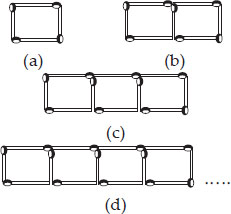(B) The given figure gives a matchstick pattern of triangles. As in Exercise 11 (A) above, find the general rule that gives the number of matchsticks in terms of the number of triangles.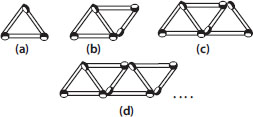SOLUTION:

(A)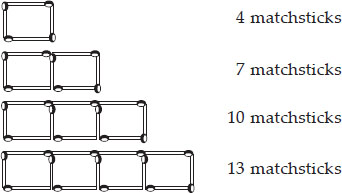If we remove 1 matchstick from each figure, then they make multiples of 3, i.e., 3, 6, 9, 12, ......
So the required equation = 3x + 1, where x is number of squares.
(B)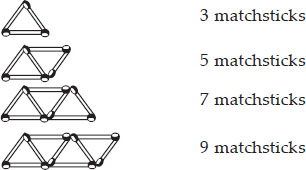If we remove 1 matchstick from each figure, then they make multiples of 2, i.e., 2, 4, 6, 8, .....
So the required equation = 2x + 1, where x is number of triangles.

Q 12.

The side of an equilateral triangle is shown by l. Express the perimeter of the equilateral triangle using l.

SOLUTION:

Side of equilateral triangle = l
Therefore, perimeter of equilateral triangle= 3 × side = 3l

Q 13.

The side of a regular hexagon (see fig.) is denoted by l.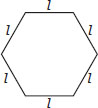Express the perimeter of the hexagon using l.
(Hint : A regular hexagon has all its six sides equal in length.)

SOLUTION:

Side of regular hexagon = l
Therefore, perimeter of regular hexagon= 6 × side = 6l

Q 14.

A cube is a three-dimensional figure as shown in the given figure. It has six faces and all of them are identical squares. The length of an edge of the cube is given by l. Find the formula for the total length of the edges of a cube.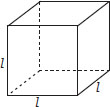SOLUTION:

Length of one edge of a cube = l
Number of edges in a cube = 12
Therefore, total length of the edges of a cube = 12 × l = 12l

Q 15.

The diameter of a circle is a line which joins two points on the circle and also passes through the centre of the circle.
(In the adjoining figure, AB is a diameter of the circle; C is its centre.) Express the diameter of the circle (d) in terms of its radius (r).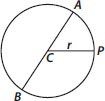SOLUTION:

Since, diameter of the circle is always twice the radius of the circle.
Therefore, d = 2r

Q 16.

To find sum of three numbers 14, 27 and 13, we can have two ways:
(A) We may first add 14 and 27 to get 41
and then add 13 to it to get the total sum 54 or
(B) We may add 27 and 13 to get 40 and then add 14 to get the sum 54.
Thus, (14 + 27) + 13 = 14 + (27 + 13)
This can be done for any three numbers. This property is known as the associativity of addition of numbers. Express this property which we have already studied in the chapter on Whole Numbers, in a general way, by using variables a, b and c.

SOLUTION:

(a + b) + c = a + (b + c), where a, b and c are any three numbers.

Q 17.

Make up as many expressions with numbers (no variables) as you can from three numbers 5, 7 and 8. Every number should be used not more than once. Use only addition, subtraction and multiplication.
(Hint : Three possible expressions are 5 + (8 - 7), 5 - (8 - 7), (5 × 8) + 7; make the
other expressions.)

SOLUTION:

(A) (8 × 5) - 7
(B) (8 + 5) - 7
(C) (8 × 7) - 5
(D) (8 + 7) - 5
(E) 5 × (7 + 8)
(F) 5 + (7 × 8)
(G) 5 + (8 - 7)
(h) 5 - (7 + 8)

Q 18.

Which out of the following are expressions with numbers only?
(A) y + 3
(B) (7 × 20) - 8z
(C) 5 (21 - 7) + 7 × 2
(D) 5
(E) 3x
(F) 5 - 5n
(G) (7 × 20) - (5 × 10) - 45 + p

SOLUTION:

(C) and (D) have expressions with numbers only.

Q 19.

Identify the operations (addition, subtraction, division, multiplication) in forming the following expressions and tell how the expressions have been formed.
(A) z + 1, z - 1, y + 17, y - 17
(B) 17y,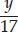, 5z
(C) 2y + 17, 2y - 17
(D) 7m, - 7m + 3, - 7m - 3

SOLUTION:

(A) z + 1 = 1 added to z
z - 1 = 1 subtracted from z
y + 17 = 17 added to y
y - 17 = 17 subtracted from y
(B) 17y = y multiplied by 17= y divided by 17
5z = z multiplied by 5
(C) 2y + 17 = First y multiplied by 2, then
2y - 17 = First y multiplied by 2, then
17 subtracted from the product
(D) 7m = m multiplied by 7
-7m + 3 = First m multiplied by -7, then 3 added to the product
-7m - 3 = First m multiplied by -7, then 3 subtracted from the product

Q 20.

Give expressions for the following cases.
(B) 7 subtracted from p
(C) p multiplied by 7
(D) p divided by 7
(E) 7 subtracted from - m
(F) - p multiplied by 5
(G) - p divided by 5
(h) p multiplied by - 5

SOLUTION:

(A) p + 7
(B) p - 7
(C) 7p
(D)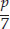(E) -m - 7
(F) -5p
(G)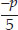(h) -5p

Q 21.

Give expressions in the following cases.
(B) 11 subtracted from 2m
(C) 5 times y to which 3 is added
(D) 5 times y from which 3 is subtracted
(E) y is multiplied by - 8
(F) y is multiplied by - 8 and then 5 is added to the result
(G) y is multiplied by 5 and the result is subtracted from 16
(h) y is multiplied by - 5 and the result is added to 16.

SOLUTION:

(A) 2m + 11
(B) 2m - 11
(C) 5y + 3
(D) 5y - 3
(E) -8y
(F) -8y + 5
(G) 16 - 5y
(h) -5y + 16

Q 22.

(A) Form expressions using t and 4. Use not more than one number operation. Every expression must have t in it.
(B) Form expressions using y, 2 and 7. Every expression must have y in it. Use only two number operations. These should be different.

SOLUTION:

(A) t + 4, t - 4, 4 - t, 4t,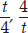(B) 2y + 7, 2y - 7, 7y + 2, 7y - 2 and so on.

Q 23.

(A) Take Sarita's present age to be y years
(i) What will be her age 5 years from now?
(ii) What was her age 3 years back?
(iii) Sarita's grandfather is 6 times her age. What is the age of her grandfather?
(iv) Grandmother is 2 years younger than grandfather. What is grandmother's age?
(v) Sarita's father's age is 5 years more than 3 times Sarita's age. What is her father's age?
(B) The length of a rectangular hall is 4 metres less than 3 times the breadth of the hall. What is the length, if the breadth is b metres?
(C) A rectangular box has height h cm. Its length is 5 times the height and breadth is 10 cm less than the length. Express the length and the breadth of the box in terms of the height.
(D) Meena, Beena and Leena are climbing the steps to the hill top. Meena is at step s, Beena is 8 steps ahead and Leena 7 steps behind. Where are Beena and Meena? The total number of steps to the hill top is 10 less than 4 times what Meena has reached. Express the total number of steps using s.
(E) A bus travels at v km per hour. It is going from Daspur to Beespur. After the bus has travelled 5 hours, Beespur is still 20 km away. What is the distance from Daspur to Beespur? Express it using v.

SOLUTION:

(A) Sarita's present age = y years
(i) After 5 years, her age will be (y + 5) years.
(ii) 3 years ago, her age was (y - 3) years.
(iii) Age of her grandfather
= 6 × (Sarita's age) = (6 × y) years
= 6y years
(iv) Age of her grandmother
= (age of her grandfather - 2) years
= (6y - 2) years
(v) Age of her father
= [3 (Sarita's age) + 5] years
= (3y + 5) years
(B) Breadth of the hall = b m
Length of the hall = [3 (breadth) - 4] m
= 3b m - 4 m = (3b - 4) m
(C) Height of the box = h cm
Length of the box = 5 × height = 5h cm
Breadth of the box = Length - 10 cm
= (5h - 10) cm
(D) Meena's position = s
Beena's position = 8 steps ahead = s + 8
Leena's position = 7 steps behind = s - 7
∴ Total number of steps = 4s - 10
(E) Speed of the bus = v km/h
Distance travelled in 5 hours = 5v km
Remaining distance = 20 km
Therefore, total distance = (5v + 20) km

Q 24.

Change the following statements using expressions into statements in ordinary language.
(For example, Given Salim scores r runs in a cricket match, Nalin scores (r + 15) runs. In ordinary language - Nalin scores 15 runs more than Salim.)
(A) A notebook costs ₹ p. A book costs ₹ 3 p.
(B) Tony puts q marbles on the table. He has 8 q marbles in his box.
(C) Our class has n students. The school has 20n students.
(D) Jaggu is z years old. His uncle is 4z years old and his aunt is (4z - 3) years old.
(E) In an arrangement of dots there are r rows. Each row contains 5 dots.

SOLUTION:

(A) A book costs 3 times the cost of a notebook.
(B) The number of marbles in Tony's box is 8 times the marbles on the table.
(C) Total number of students in the school is 20 times the number of students in our class.
(D) Jaggu's uncle is 4 times older than Jaggu and Jaggu's aunt is 3 years younger than his uncle.
(E) The total number of dots is 5 times the number of rows.

Q 25.

(A) Given Munnu's age to be x years, can you guess what (x - 2) may show?
(Hint : Think of Munnu's younger brother.)
Can you guess what (x + 4) may show? What (3x + 7) may show?
(B) Given Sara's age today to be y years. Think of her age in the future or in the past.
What will the following expression indicate? y + 7, y - 3, y + 4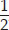, y - 2.
(C) Given n students in the class like football, what may 2n show? What may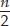show? (Hint : Think of games other than football).

SOLUTION:

(A) Munnu's age = x years
Age of his younger brother who is
2 years younger than him = (x - 2) years
Age of his elder brother who is 4 years elder than him = (x + 4) years
Age of his father whose age is 7 years more than thrice of his age = (3x + 7) years
(B) Sara's present age = y years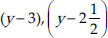represents Sara's age in past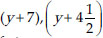represents Sara's age in future
(C) Number of students who like football = n
Number of students who like hockey is twice the number of students who like football, i.e., 2n
Number of students who like tennis is half the number of students who like football, i.e.,Q 26.

State which of the following are equations (with a variable). Give reason for your answer. Identify the variable from the equations with a variable.
(A) 17 = x + 7
(B) (t - 7) > 5
(C)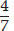= 2
(D) (7 × 3) - 19 = 8
(E) 5 × 4 - 8 = 2x
(F) x - 2 = 0
(G) 2m < 30
(h) 2n + 1 = 11
(i) 7 = (11 × 5) - (12 × 4)
(j) 7 = (11 × 2) + p
(k) 20 = 5y
(l)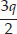< 5 (m) z + 12 > 24
(n) 20 - (10 - 5) = 3 × 5 (o) 7 - x = 5

SOLUTION:

(A) 17 = x + 7 is an equation with variable as both the sides are equal. The variable is x.
(B) (t - 7) > 5 is not an equation as L.H.S. is greater than R.H.S.
(C)= 2 is not an equation with variable.
(D) (7 × 3) - 19 = 8 is not an equation with variable.
(E) 5 × 4 - 8 = 2x is an equation with variable as both the sides are equal. The variable is x.
(F) x - 2 = 0 is an equation with variable as both the sides are equal. The variable is x.
(G) 2m < 30 is not an equation as L.H.S. is less than R.H.S.
(h) 2n + 1 = 11 is an equation with variable as both the sides are equal. The variable is n.
(i) 7 = (11 × 5) - (12 × 4) is not an equation with variable.
(j) 7 = (11 × 2) + p is an equation with variable as both the sides are equal. The variable is p.
(k) 20 = 5y is an equation with variable as both the sides are equal. The variable is y.
(l)< 5 is not an equation as L.H.S. is less than R.H.S.
(m) z + 12 > 24 is not an equation as L.H.S. is greater than R.H.S.
(n) 20 - (10 - 5) = 3 × 5 is not an equation with variable.
(o) 7 - x = 5 is an equation with variable as both the sides are equal. The variable is x.

Q 27.

Complete the entries in the third column of the table.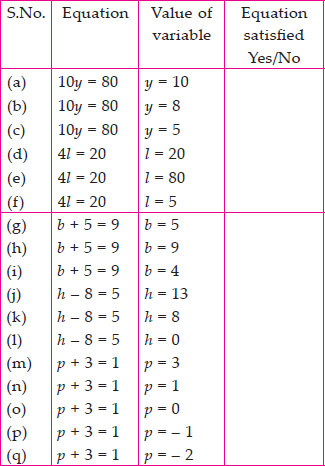SOLUTION: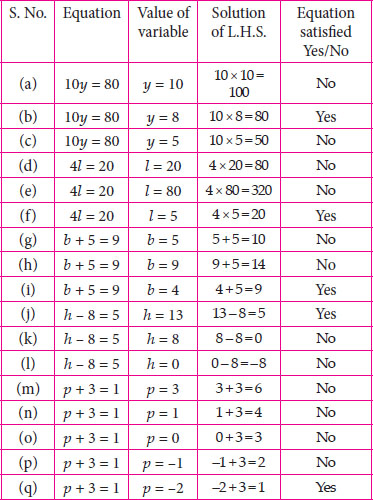Q 28.

Pick out the solution from the values given in the bracket next to each equation. Show that the other values do not satisfy the equation.
(A) 5m = 60 (10, 5, 12, 15)
(B) n + 12 = 20 (12, 8, 20, 0)
(C) p - 5 = 5 (0, 10, 5 - 5)
(D)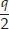= 7 (7, 2, 10, 14)
(E) r - 4 = 0 (4, - 4, 8, 0)
(F) x + 4 = 2 (- 2, 0, 2, 4)

SOLUTION:

(A) 5m = 60
Putting the given values in L.H.S., we get
5 × 10 = 50
∵ L.H.S. ≠ R.H.S.
m = 10 is not the solution.
5 × 5 = 25
L.H.S. ≠ R.H.S.
m = 5 is not the solution.
5 × 12 = 60
∵ L.H.S. = R.H.S.
m = 12 is a solution.
5 × 15 = 75
∵ L.H.S. ≠ R.H.S.
m = 15 is not the solution.
(B) n + 12 = 20
Putting the given values in L.H.S., we get
12 + 12 = 24
L.H.S. ≠ R.H.S.
n = 12 is not the solution.
8 + 12 = 20
∵ L.H.S. = R.H.S.
n = 8 is a solution.
20 + 12 = 32
∵ L.H.S. ≠ R.H.S.
n = 20 is not the solution.
0 + 12 = 12
∵ L.H.S. ≠ R.H.S.
n = 0 is not the solution.
(C) p - 5 = 5
Putting the given values in L.H.S., we get
0 - 5 = -5
∵ L.H.S. ≠ R.H.S.
p = 0 is not the solution.
10 - 5 = 5
∵ L.H.S. = R.H.S.
p = 10 is a solution.
5 - 5 = 0
∵ L.H.S. ≠ R.H.S.
p = 5 is not the solution.
-5 - 5 = -10
L.H.S. ≠ R.H.S.
p = -5 is not the solution.
(D)= 7
Putting the given values in L.H.S., we get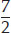= 3.5
∵ L.H.S. ≠ R.H.S.
q = 7 is not the solution.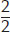= 1
∵ L.H.S. ≠ R.H.S.
q = 2 is not the solution.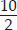= 5
∵ L.H.S. ≠ R.H.S.
q = 10 is not the solution.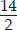= 7
∵ L.H.S. = R.H.S.
q = 14 is a solution.
(E) r - 4 = 0
Putting the given values in L.H.S., we get
4 - 4 = 0
∵ L.H.S. = R.H.S.
r = 4 is a solution.
-4 - 4 = -8
∵ L.H.S. ≠ R.H.S.
r = -4 is not the solution.
8 - 4 = 4
∵ L.H.S. ≠ R.H.S.
r = 8 is not the solution.
0 - 4 = -4
∵ L.H.S. ≠ R.H.S.
r = 0 is not the solution.
(F) x + 4 = 2
Putting the given values in L.H.S., we get
-2 + 4 = 2
∵ L.H.S. = R.H.S.
x = -2 is a solution.
0 + 4 = 4
∵ L.H.S. ≠ R.H.S.
x = 0 is not the solution.
2 + 4 = 6
∵ L.H.S. ≠ R.H.S.
x = 2 is not the solution.
4 + 4 = 8
∵ L.H.S. ≠ R.H.S.
x = 4 is not the solution.

Q 29.

(A) Complete the table and by inspection of the table, find the solution to the equation m + 10 = 16.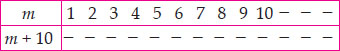(B) Complete the table and by inspection of the table, find the solution to the equation 5t = 35.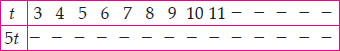(C) Complete the table and find the solution of the equation z/3 = 4 using the table.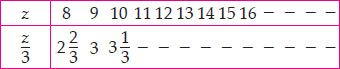(D) Complete the table and find the solution to the equation m - 7 = 3.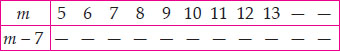SOLUTION:

(A)
 m 1 2 3 4 5 6 7 8 9 10 11 12 13 m + 10 11 12 13 14 15 16 17 18 19 20 21 22 23

∵ At m = 6, m + 10 = 16
m = 6 is the solution.
(B)
 t 3 4 5 6 7 8 9 10 11 12 13 14 15 16 5t 15 20 25 30 35 40 45 50 55 60 65 70 75 80

∵ At t = 7, 5t = 35
t = 7 is the solution.
(C)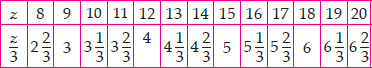∵ At z = 12,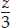= 4
z = 12 is the solution.
(D)
 m 5 6 7 8 9 10 11 12 13 14 15 m - 7 -2 -1 0 1 2 3 4 5 6 7 8

∵ At m = 10, m - 7 = 3
m = 10 is the solution.

Q 30.

Solve the following riddles, you may yourself construct such riddles.
Who am I?
(i) Go round a square
Counting every corner
Thrice and no more!
To get exactly thirty four!
(ii) For each day of the week
Make an upcount from me
If you make no mistake
You will get twenty three!
(iii) I am a special number
Take away from me a six!
A whole cricket team
You will still be able to fix!
(iv) Tell me who I am
I shall give a pretty clue!
You will get me back
If you take me out of twenty two!

SOLUTION:

(i) According to given information, we have
3(4) + x = 34 ⇒ 12 + x = 34
x = 34 - 12 ⇒ x = 22
(ii) According to given information, we have
x + 7 = 23
x = 23 - 7 ⇒ x = 16
(iii) According to given information, we have
x - 6 = 11
x = 11 + 6 ⇒ x = 17
(iv) According to given information, we have
x = 22 - x x + x = 22 ⇒ 2x = 22
x =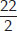x = 11